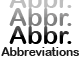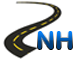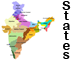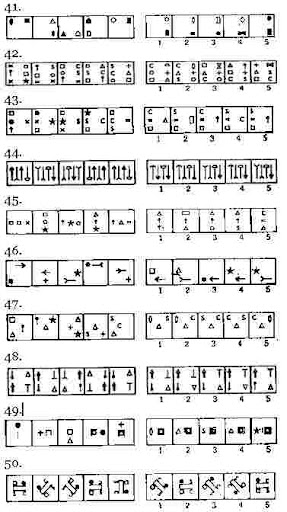Reasoning Part 1 -List of AbbreviationsNational Highways in IndiaStates & Union Territories of IndiaCurrency of All Countries

# Reasoning Part 1

1. In a certain code ROPE is written as \$3%6 and RITE is written as \$4#6. How is PORT written in that code?

(1) %4\$#

(2) \$3%#

(3) \$64%

(4) %3\$#

(5) None of these

2. How many such pairs of letters are there in the word KNIGHT, each of which has as many letters between them in the word as they have in the English alphabet?

(1) None

(2) One

(3) Two

(4) Three

(5) More than three

3. Pointing to a photograph Nikita said ‘She is the only grad daughter of my grandmother’s daughter’. How is the girl in photograph related to Nikita?

(1) Sister

(2) Niece/ daughter

(3) Aunt

(4) Cannot be determined

(5) None of these

4. Four of the following five are alike in a certain way and so form a group. Which is the one that does not belong to the group?

(1) Pineapple

(2) Guava

(3) Grapes

(4) Papaya

(5) Pear

5. If the word STABLE all the consonants are replaced by the previous letter and all the vowels are replaced by the next letter which letter will be third from the left end?

(1) S

(2) B

(3) A

(4) K

(5) None of these

6. In a certain code HOUSE is written as FTVPI, how is CHAIR written in that code?

(1) DIBJS

(2) SBJID

(3) SHBGD

(4) SJBID

(5) None of these

7. Four of the following five are alike in a certain way and so form a group. Which is the one that does not belong to the group?

(1) FH

(2) KM

(3) PR

(4) CE

(5) JM

8. If ‘ P Q’ means ‘P is wife of Q’, ‘P+Q’ means ‘P is father of Q’ and ‘P Q’ means ‘P is sister of Q’ then in G H +R D, how is G related to D?

(1) Cannot be determined

(2) Mother

(3) Niece

(4) Aunt

(5) None of these

9. If the digits of the number 5726489 are arranged in ascending order, how many digits will remain at the same position?

(1) None

(2) One

(3) Two

(4) Three

(5) More than three

10. Four of the following five are alike in a certain way and so form a group. Which is the one that does not belong to the group?

(1) 17

(2) 19

(3) 23

(4) 29

(5) 27

11. How many meaningful words can be made from the letters AEHT, using each letter only once?

(1) None

(2) One

(3) Two

(4) Three

(5) More than three

12. If ‘’ means ‘x’, \$ means ‘+’, # means ‘ ‘ and ‘©’ means ‘-‘ then what is the value of

360 # 24\$ 56« 5© 48?

(1) 253

(2) 242

(3) 247

(4) 285

(5) None of these

13. If blue means pink, pink means green, green means yellow, yellow means red and red means white then what is the colour of turmeric?

(1) green

(2) yellow

(3) red

(4) pink

(5) None of these

14. If it is possible to make a meaningful word from the second, the third, the tenth and the eleventh letters of the word PASSIONATELY using each letter only once, second letter of that word is your answer. If no such word can be formed your answer is X and if more than one word can be formed your answer is Y.

(1) A

(2) E

(3) L

(4) X

(5) Y

15. In a row of children facing north Manish is fourth to the left of Suresh who is tenth from the left end. Nisha is second to the right of Suresh and eighth from the right end of the row. Total how many children are there in the row?

(1) 19

(2) 20

(3) 21

(4) 18

(5) None of these

Directions (16-20): In these questions symbols ©, #, «, \$ and @ are used with different meanings as follows:

‘A © B’ means ‘A is smaller than B’

‘A # B’ means ‘A is either smaller than or equal to B’

‘A « B’ means ‘A is greater than B’

‘A \$ B’ means ‘A is either greater than or equal to B’

‘A @ B’ means ‘A is neither smaller than nor greater than B’

In each of the following questions assuming the given statements to be true, find out which of the two conclusions I and II given below them is/ are definitely true

Give answer (1) if only conclusion I is true.

Give answer (2) if only conclusion II is true.

Give answer (3) if either conclusion I or conclusion II is true.

Give answer (4) if neither conclusion I nor conclusion II is true.

Give answer (5) if both conclusions I and II are true.

Conclusions: I. V © L

II. S © J

17. Statements: M#R, R©J, J#H

Conclusions: I. M #H

II. R © H

18. Statements: H\$F, F@G, G«M

Conclusions: I. H «M

II. H «G

19. Statements: R©J, J «T,,T # L

Conclusions: I. R @ T

II. J @ l

20. Statements: W@T, T\$K, K «F

Conclusions: I. W \$ K

II. W @ K

Directions (21-25): In each question below there are three statements followed by two conclusions numbered I and II. You have to take the three given statements to be true even if they seem to be at variance from commonly known facts and then decide which of the given conclusions logically follows from the three given statements disregarding commonly known facts. Then decide which of the answers (1), (2), (3), (4) and (5) is the correct answer and indicate it on the answer sheet.

Give answer (1) if only conclusion I is follows.

Give answer (2) if only conclusion II is follows

Give answer (3) if either conclusion I or II follows.

Give answer (4) if neither I nor II is follows.

Give answer (5) if both I and II follow.

21. Statements:

Some pens are books.

All books are pencils.

All pencils are jars.

Conclusions:

I. All books are jars.

II. Some pens are pencils.

22. Statements:

Some bowls are spoons

Some spoons are forks

All forks are plates.

Conclusions:

I. Some bowls are forks.

II. Some spoons are plates.

23. Statements:

Some bottles are jars.

All jars are buckets.

All buckets are tanks.

Conclusions:

I. All jars are tanks.

II. Some buckets are tanks.

24. Statements:

Some phones are mobiles.

Some mobiles are computers.

Some computers are keys.

Conclusions:

I. Some phones are keys.

II. Some computes are phones.

25. Statements:

All papers are files.

Some files are folders.

All folders are bags.

Conclusions:

I. Some files are bags.

II. Some papers are folders.

Directions (26-30): Each of the questions below consists of a question and two statements numbered I and II are given below it. You have to decide whether the data provided in the statements are sufficient to answer the question. Read both the statements and

Give answer (1) if the data is Statement I alone are sufficient to answer the question, while the data in Statement II alone are not sufficient to answer the question.

Give answer (2) if the data in Statement II alone are sufficient to answer the question, while the data in Statement I alone are not sufficient to answer the question.

Give answer (3) if the data in Statement I alone or in Statement II alone are sufficient to answer the question.

Give answer (4) if the data in both the Statement I and II are not sufficient to answer the question.

Give answer (5) if the data in both the Statement I and II together are necessary to answer the question.

26. Lalita is in which direction with respect to Sangita?

I. Lalita is to the East of Prabha who is to the South of Sangita.

II. Vinita is to the North of Lalita who is to the East of Sangita.

27. What is the code for ‘Play’ in the code language?

I. In the code language ‘play and dance’ is written as ‘ka to pe’

II. In the code language enjoy the dance’ is written as ‘pe jo ra’.

28. How many children are there in the class?

I. Vandana’s rank in the class is five ranks below Nandini who is twenty fifth from the bottom.

II. Nandini’s rank is seventeenth from the top.

29. Who is tallest among Neeta, Sudha, Radha, Maya and Geeta?

I. Radha is shorter than Neeta and Sudha but not shorter than Maya and Geeta.

II. Neeta is not the tallest.

30. How many sons does Ramesh have?

I. F is sister of H who is son of Ramesh.

II. R is brother of H.

Directions (31-35): These questions are based on the following letter / number/ symbol arrangement . Study it carefully and answer the questions that follow:

5 D G E « 7 9 \$ F 1 6 R % L I A J 3 B # 4 @ K P 8 U M 2

31. Four of the following five are alike in a certain way on the basis of their positions in the above arrangement and so form a group. Which is the one that does not belong to the group?

(1) \$9F

(2) R6%

(3) 8PU

(4) #B4

(5) 3BJ

32. If all the symbols are removed from the above arrangement which element will be third to the left of thirteenth from the left?

(1) L

(2) R

(3) 6

(4) I

(5) None of these

33. What will come in place of the question mark (?) in the following series based on the above arrangement?

EDH 9 « 7 I\$F ?

(1) 6RI

(2) %R6

(3) R16

(4) %6R

(5) None of these

34. Which element will be fifth to the right of ninth from the right end if all the numbers are removed from the above arrangement?

(1) K

(2) @

(3) P

(4) #

(5) None of these

35. How many such numbers are there in the above arrangement each of which is immediately followed by a consonant but not immediately preceded by a vowel?

(1) None

(2) One

(3) Two

(4) Three

(5) More than three

Directions (36-40): These questions are based on the basis of following information. Study it carefully and answer the questions.

Eight executives J, K, L, M, N, O, P, and Q are sitting around a circular table for a meeting. J is second to the right of P who is third to the right of K. M is second to the left of O who sits between P and J, L is not a neighbour of K or N.

36. Who is to the immediate left of L?

(1) Q

(2) O

(3) K

(4) N

(5) None of these

37. Who is to the immediate left of K?

(1) N

(2) J

(3) Q

(4) Cannot be determined

(5) None of these

38. Which of the following is the correct position of N?

(1) Second to the right of K

(2) To the immediate left of K

(3) To the immediate right of M

(4) To the immediate right of K

(5) None of these

39. Who is third to the right of P?

(1) L

(2) J

(3) Q

(4) N

(5) None of these

40. Which of the following groups of persons have the first person sitting between the other two?

(1) PJO

(2) OPJ

(3) OPM

(4) MPO

(5) None of these

Directions (41-50): In each of the questions given below which one of the five answer figures on the right should come after the problem figures on the left, if the sequence were continued?Click Here to Find Latest Jobs and Current Affairs